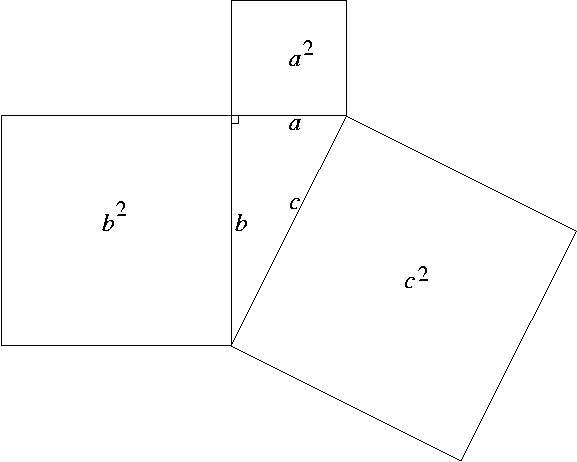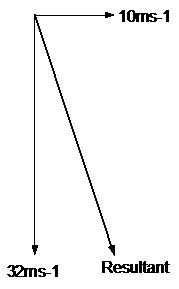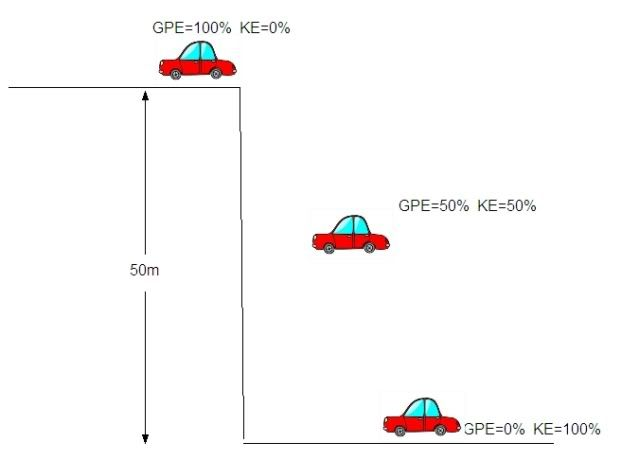Equations unpacked and explained

Study matter and its motion through spacetime...

Moderators: kiore, Blip, The_Metatron

Inspired by discussion in the 'Latex on Ratskep' thread. I thought it would be a good idea to have a thread in which we unpack some of the core equations in physics and explain what they mean. The aim of this is to aid people in understanding mathematical notation (myself included, as this is probably the area in which my understanding is most limited), to get us used to reading the language (probably the thing that puts many off), and to make good use of the new toys that LIFE has provided us. To keep this thread tidy, I will start a separate thread for discussion, which will include elucidation of any errata.

To begin, just a little background on what equations are and how they work:

So just what is an equation? Well, at bottom, it's simply a description of a relationship. Let's look at the simplest equation:

Well, this all looks very straightforward, doesn't it? It's fairly simple to see what is happening here. The important bit of that is the sign, which denotes equality. What is on one side of that sign will always be exactly equal to what is on the other side. They are being equated! So the two sides of that sign are directly equivalent.

But what about the terms? Well, in this simple equation, all the terms are variables. The sign tells us that where one of the terms on one side is varied, the term on the other side will also vary to compensate. So, for example, if we vary the integers in the input, we will get a different output to reflect this:

And indeed we can generate another equation that shows that, however the input is varied, the output will always give an equality.

Where is any number and where denotes the sum.

Now a look at another familiar equation. We're all (I hope) familiar with Pythagoras' Theorem. It provides a means for calculating the length of one side of a right-angled triangle in Euclidean geometry given the lengths of two sides as input. Formally stated, it tells us that the square of the hypotenuse of a right-angled triangle is equal to the sum of the squares on the other two sides. It looks like this:In the notation, this would be . Thus, if we take to be 3 and to be 6, we can carry out the calculation using those two lengths as input:

and , so we sum those two figures to obtain . Taking that, we find the square root of 45 , which gives us a length for the hypotenuse of .

We can also carry this out backwards, by taking, for example, and as input. If we know that , and that , we can calculate what will be. and , so we can calculate this by subtraction: . Now we need the square root of 60 , and we now know that .

7.7 what, you might ask? Well, as long as the units of measurement are consistent, this doesn't matter. By consistent, we mean that either they are expressed in the same units both sides of the equation or that suitable conversions have been carried out.

Now, all of the above is working with variable terms. In physics, the really interesting things happen when we introduce the notion of a constant. Much of physics can be identified with the finding of constant quantities, because these tell us the most interesting things in physics, not least because constants are often the conversion rates between pairs of variables. Take the most famous equation in physics as an example, Einstein's famous mass/energy relationship.

What this equation tells us is that the energy contained in a given amount of mass is equal to the mass multiplied by the square of the speed of light. In order to unpack it, we need to define the terms properly and give the units in which the terms are measured.

denotes the energy, and this is measured in joules. A joule is defined as the energy expended in applying a force of 1 newton through 1 metre.
denotes the mass, and is measured in Kilograms*.
denotes the speed of light in a vacuum, and this is measured in metres per second. The actual value of this is , but for simplicity, we'll take it to be , or or .

So, let's plug in some numbers (actually, just 1 number, namely the mass). Let's take our mass to be 1 Kg for simplicity. First, though, we need to square the speed of light in a vacuum. So, we get which gives us , which we multiply again by 1 Kg, which gives us . This last result can be converted directly to joules, as the units are given in metres per second. From this, we obtain the result that the energy contained in a mass of 1 Kg, is equal to joules, or 90,000,000,000,000,000 joules.

This is a huge number. What this tells us is that the energy in a single standard bag of sugar is roughly equivalent to the output of 21.5 million tons of TNT. Putting that into perspective, Little Boy, the bomb dropped on Hiroshima in 1945, had an energy yield of 15,000 tons of TNT.

*Although we are using a term denoting weight in everyday terms, weight is simply the effect of gravity on mass, so in everyday terms, the weight of 1 Kg is the mass of 1 Kg at 1G gravity, the latter being gravity as measured on Earth. So the Kg is a robust measure of mass as long as it is understood that we aren't actually talking about weight, but mass.
Last edited by hackenslash on Feb 14, 2012 5:09 pm, edited 1 time in total.
hackenslash

Name: The Other Sweary One
Posts: 22910
Age: 53Country: Republic of Mancunia
Print view this post

I will talk today about simple physics. That is position, velocity and acceleration:

The above two equations give us the position r(t) and velocity v(t) of any object at a time t, starting with at a position x0, with velocity v0 and acceleration a. The (t) means that the position r and velocity v are functions of time t.

The above equations of course are for one dimensional trajectories. However, one can use the above equations as starting points for two and three-dimensional motion.

For example we have a car that starts with velocity v0 = 0, from position x0= 0, and arrives at position r(t) = 10 m in 5 seconds. What is the acceleration a? And what are the two equations?

Plugging in the numbers in the first equation, we get:

in simple mathematical terms, that velocity is the rate of change of position and can be derived from any equation of position; similarly:

Acceleration is the rate of change of velocity, and so can be derived from the equation for velocity.

Take care to distinguish between velocity and speed in physics; they are not the same. Speed in physics is purely the number like for example 1 m s; but velocity is both speed and direction, so velocity can also be negative. Same is with acceleration; in physics even deceleration or a constant change in velocity's direction is considered to be acceleration.

This is important when considering a pendulum for example and its motion; or generally sinusoidal motion:

Where, in this case, I consider b and ω constants.

Then we can derive the velocity of the above by differentiation:

and differentiating velocity we can get to acceleration:Darkchilde
RS Donator

Posts: 9015
Age: 53Country: United KingdomPrint view this post

Here's the sort of problem I give to my students in their 'Introduction to Mechanics' section (a bit of GCSE/AS physics crossover). To solve the problem we use the bit of physics and maths introduced by Hack and Darkchilde.

"MrC foolishly drives his 1981 Volvo estate car off a cliff. The car is intially moving with only a horizontal speed of 10ms-1. The cliff is 50m high. At what speed will he meet his impending death?
(assume that there is no air resistance and take g=10ms-2)"

To solve this problem we need to split the motion of the car into two components; the horizontal and the vertical.
Forces in the vertical direction (gravity) will not affect motion in the horizontal (where we have assumed no forces).

First of all, the equations of motion can can written as;

1) *
2)
3) *

where;

S=distance travelled
u=initial velocity
v=final velocity
a=acceleration
t=time

*note that equation 1) and 3) are just versions of the ones Darkchilde wrote.

Solving the vertical component of velocity

The car starts off with an initial vertical velocity of u=0ms-1, we also know that it is accelerating at a=10ms-2 and the distance it will fall is S=50m.

We want to find the cars final velocity as it hits the ground below, so we use:

and root both sides to find ;

then substitute the values for u,a and S;

(nearest whole number)

Combining Horizontal and Vertical components

So, we now have the vertical velocity at which the car hits the bottom.
Recall that we already now the horizontal velocity at which the car meets the ground, it will be 10ms-1 because horizontally we have ignored air resistance i.e. the car won't slow down / speed up in that direction.

To find the speed that the car hits, we need to combine the horizontal and vertical components using a bit of pythagorus. The resultant speed will be the hypotenuse of the right angled triangle with sides 10ms-1 and 32ms-1.So, I meet my death at a speed of 34ms-1edit - for spelling! Also, I've been a bit 'cavalier' with the terms 'velocity' and 'speed'. To be clear; Velocity is the Speed in a stated direction.Scarlett and Ironclad wrote:Campermon,...a middle aged, middle class, Guardian reading, dad of four, knackered hippy, woolly jumper wearing wino and science teacher.campermon
RS Donator

Posts: 17443
Age: 52Print view this post

Further to my previous post, here's another way to solve the vertical final velocity.
Here's the problem again;

"MrC foolishly drives his 1981 Volvo estate car of a cliff. The car is intially moving with only a horizontal velocity of 10ms-1.The cliff is 50m high. At what speed will he meet his impending death?
(assume that there is no air resistance and take g=10ms-2)"

This time, instead of using the equations for motion, I'll use the idea that energy is conserved. A typical textbook definition being;

"Energy cannot be created or destroyed, it can only be transferred from one type to another."

The energies we are concerned with here are;

Gravitational Potential Energy (Ep) and Kinetic Energy (Ek), given by:

where m=mass(Kg), g=acceleration due to gravity(ms-2), h=height(m) and v=velocity(ms-1)

We're going to ignore the horizontal motion for the time being and just imagine the car starting at the top of the cliff (with a verical initial velocity of 0ms-1) and then falling to hit the ground with some velocity v.

So, in this case, the car starts off with no KE and has only GPE. As the car falls the GPE is transferred to KE. At exactly half way down the car has 50% GPE and 50% KE. At the bottom of the cliff all of the GPE it started with has been transferred to KE. (see diagram):In words, the gravitational energy we started with is equal to the kinetic energy we ended with. Mathematically;

and substituting the formulae;

Canceling out the mass on each side;

and rearranging to make v the subject;

Note that in the previous post we got;

with u=0, so

This is exactly the same solution. The only difference being the choice of symbols (both g and a are accelerations, both h and S are distances).

if we plug in the numbers we'll get the same answer for the vertical final velocity. This is why physics is so cool - we approach the same problem with two different methods and get the same result!

This is no coincidence! To understand why, you'll have to wait for Darkchildes calculus primer and use the results to understand where we get the formulae for energy from!Scarlett and Ironclad wrote:Campermon,...a middle aged, middle class, Guardian reading, dad of four, knackered hippy, woolly jumper wearing wino and science teacher.campermon
RS Donator

Posts: 17443
Age: 52Print view this post

This has come up elsewhere on the forum, so I thought it might be fun to pull apart The Drake Equation.

So what is the Drake Equation? Well, it's actually more like a thought experiment designed to show what information we would need to robustly calculate the number of communicating civilisations in our galaxy. The equation is made up almost entirely of unknown variables. It's formulated as follows:

Where:
= the number of civilizations in our galaxy that may be communicating

= the average rate of star formation per year in our galaxy

= the percentage of those stars that have planets

= the average number of planets that can potentially support life per star that has planets

= the percentage of the above where life independently evolves

= the percentage of those where life develops intelligence

= the percentage of civilizations that develop a technology that can be detected

= the length of time such civilizations release detectable signals into space

As we can see, all the variables in this equation are unknown, with only the exception of , for which we can generate a reasonably robust figure, although not that robust, in reality. We can also, in light of recent observations, begin to put a figure to .It may be that, as time moves on, we can put figures on some of these variables. At the moment, however, they remain 'guesstimates'. So, here's how it works in practice.

If we take our starting figure for the number of stars in our galaxy as 300 billion (it's actually estimated at somewhere between 200 and 400 billion, so this figure will serve well enough for our purposes here) Then:

(approx)
(approx)

After this, things get really woolly, and we can only really guess. No matter, we'll take those two as our starting figure and put in some estimates for the other values. So let's assume that:

(based on the only example we know of)
(wild stab)
(wild stab)
(wild stab)
(wild stab: We hope it will be at least this, but we only have one example to go on, and an asteroid - among other things - could seriously curtail that figure)

The output here would be 1,200. That means that, based on this equation with those figures as input, there should be about 1,200 communicating civilisations in our galaxy right now. That looks like a pretty big figure, but that's peanuts to space (RIP DA). Our galaxy is 100,000 light years across. Via , that gives us an area for the galaxy of 7,850,000,000 square light-years. Dividing this by the number of communicating civilisations in the galaxy (assuming a uniform distribution), we get an average distance between communicating civilisations of approximately 7,000 light-years.

Now, given that we have been transmitting radio for about 70 years, give or take, and that radio signals propagate at , this means that, on average, out signals will have made it approximately 1% of the distance to the nearest civilisation. Similarly, if we were actually to have received a signal from a communicating civilisation, that signal would have to have left its source at precisely the right 70 year window 7,000 years ago to arrive during the window of time in which we've been able to detect such signals, again the last 70 years (actually slightly less, because we haven't been using radio for that purpose for that long).

It should also be noted that if any of those variables in the Drake equation tend toward zero, which is a very real possibility, then that output goes down dramatically, meaning that the average distance between communicating civilisations goes up just as dramatically. Indeed, it is far from being beyond the realm of possibility that we are the first communicating civilisation in the galaxy. If we were to rigorously deal with some of those variables above, we'd quite probably find that our estimates are too generous. Intelligence isn't the only requirement for a communicating civilisation, after all. Opposable thumbs (or some equivalent trait), curiosity, motivation, etc, are all factors in what ultimately looks like.

I hope that the above clarifies what the Drake equation's limitations are, and why the Fermi paradox isn't a paradox.

Thanks to Darkchilde for her help with some of the numbers.Oh, and to Pulsar for his excellent tutorial, upon which I leaned significantly.hackenslash

Name: The Other Sweary One
Posts: 22910
Age: 53Country: Republic of Mancunia
Print view this post

Presumably the Drake Equation is subject to
alteration if any new data comes into existence
But it really is just an elaborate conjecture at best
and shaII probably in aII certainty never be verified or
confirmed which in part invalidates it : need to get used
to the idea that we will never know everything and this is one
example of that : the best way to proceed with any unaswerable
question is to maintain a neutral stance by referencing a degree of
open mindedness and not to become bogged down in maths : that deals
with what is true and not with what may be true : as after aII probability is
a tricky business : so easy then every gambling institution on the planet would
be bankrupt by now but last time I checked Las Vegas was still open for business
A MIND IS LIKE A PARACHUTE : IT DOES NOT WORK UNLESS IT IS OPEN
surreptitious57

Posts: 10203

Print view this post